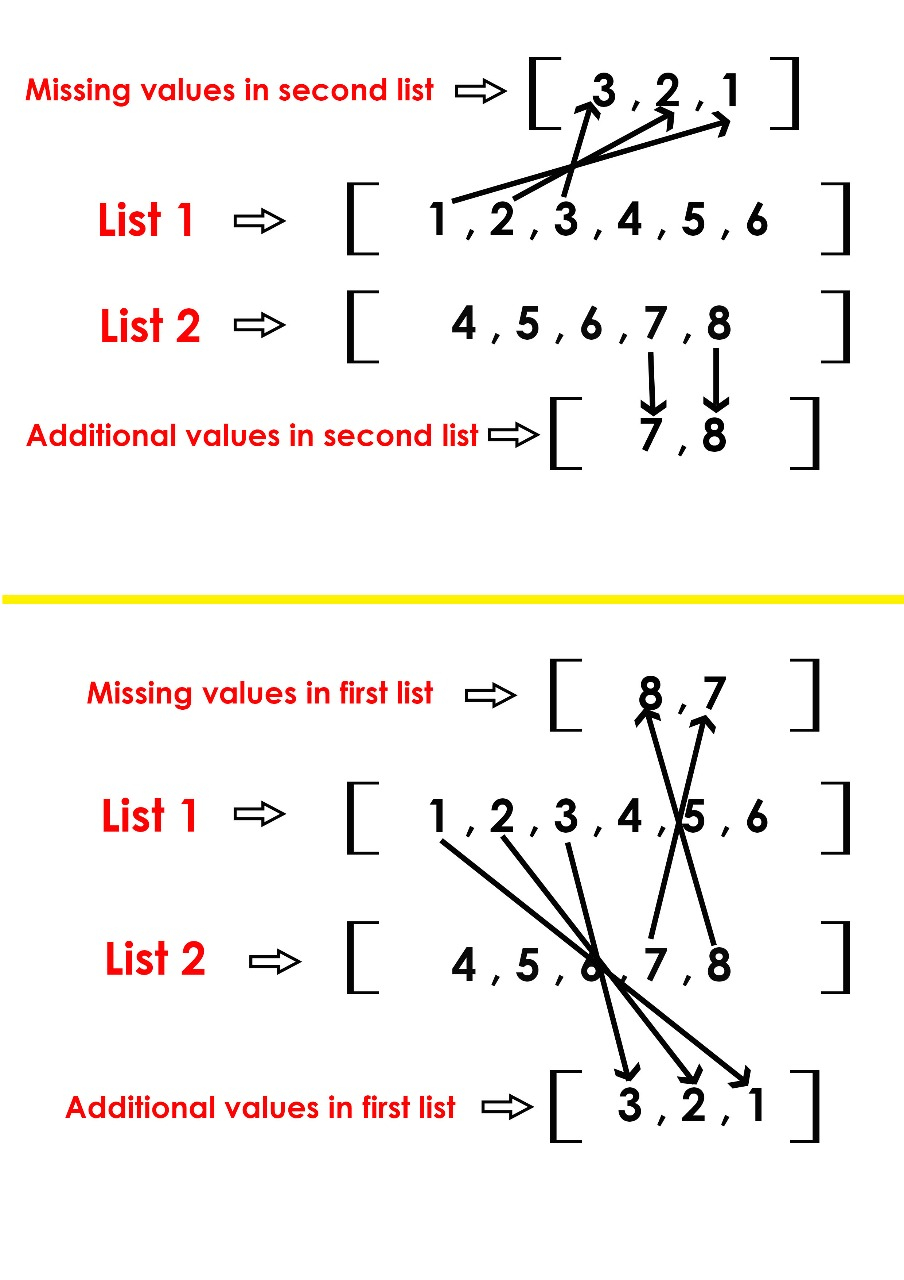Python | Find missing and additional values in two lists

• Difficulty Level : Easy
• Last Updated : 21 Nov, 2018

Given two lists, find the missing and additional values in both the lists.

Examples:

Input : list1 = [1, 2, 3, 4, 5, 6]
list2 = [4, 5, 6, 7, 8]
Output : Missing values in list1 = [8, 7]
Additional values in list1 = [1, 2, 3]
Missing values in list2 = [1, 2, 3]
Additional values in list2 = [7, 8]

Explanation:Recommended: Please try your approach on {IDE} first, before moving on to the solution.

Approach: To find the missing elements of list2 we need to get the difference of list1 from list2. To find the additional elements of list2, calculate the difference of list2 from list1.
Similarly while finding missing elements of list1, calculate the difference of list2 from list1. To find the additional elements in list1, calculate the difference of list1 from list2.

Insert the list1 and list2 to set and then use difference function in sets to get the required answer.

Prerequisite : Python Set Difference

 # Python program to find the missing # and additional elements   # examples of listslist1 = [1, 2, 3, 4, 5, 6]list2 = [4, 5, 6, 7, 8]  # prints the missing and additional elements in list2 print("Missing values in second list:", (set(list1).difference(list2)))print("Additional values in second list:", (set(list2).difference(list1)))  # prints the missing and additional elements in list1print("Missing values in first list:", (set(list2).difference(list1)))print("Additional values in first list:", (set(list1).difference(list2)))

Output:

Missing values in second list: {1, 2, 3}
Additional values in second list: {7, 8}
Missing values in first list: {7, 8}
Additional values in first list: {1, 2, 3}
My Personal Notes arrow_drop_up